## May 29, 2011

### PageRank is an abomination (mathematically)

Filed under: data analysis,mathematics,rants,reviews — Peter Saveliev @ 10:27 am

My recent interest in data analysis has lead me to read about its uses in Internet search, then to Google search algorithm, then finally to its core, the PageRank.

PageRank is a way to compute the importance of web-pages based on their incoming and outgoing links.

PageRank is a mathematical idea that “made” Google’s web search algorithm. In fact, PageRank was the search algorithm, initially. Even with so many things added, PageRank remains the cornerstone of web search. Therefore, its problems become problems of web search and, hence, our problems. These problems, I believe, are mathematical in nature.

As a mathematical idea I find PageRank very unsatisfying. The reason is that PageRank, as well its derivatives, uses made-up parameters.

## Probabilistic interpretation of PageRank

Let’s recall the basics of PageRank.

According to Google’s own Matt Cutts (the pictures come from the original paper):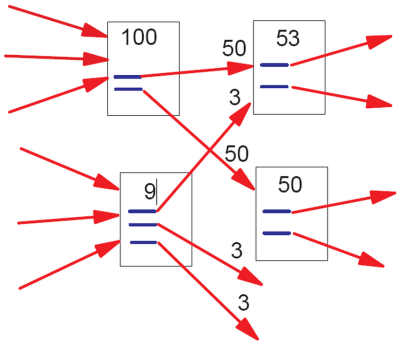“In the image above, the lower-left document has “nine points of PageRank” and three outgoing links. The resulting PageRank flow along each outgoing link is consequently nine divided by three = three points of PageRank.

That simplistic model doesn’t work perfectly, however. Imagine if there were a loop: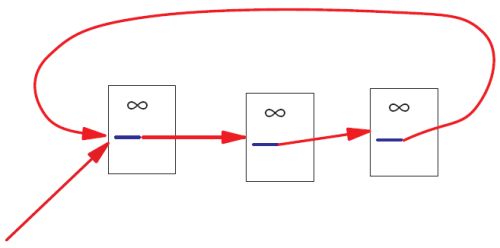No PageRank would ever escape from the loop, and as incoming PageRank continued to flow into the loop, eventually the PageRank in that loop would reach infinity. Infinite PageRank isn’t that helpful [smiley face] so Larry and Sergey introduced a decay factor–you could think of it as 10-15% of the PageRank on any given page disappearing before the PageRank flows along the outlinks. In the random surfer model, that decay factor is as if the random surfer got bored and decided to head for a completely different page.”

Seems like a reasonable model?

Reading about the “decay factor” trick made me laugh. I am imagining the two Google guys thinking: “OK, we are getting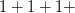$1+1+1+$
But it goes to infinity! What to do, what to do? Eureka! Let’s make the sequence into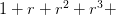$1+r+r^2+r^3+$…,
i.e., a geometric progression!” (Even though this step is mathematically silly, I have to admit that the explanation, “the bored surfer”, is brilliant! Post hoc?)

Now, what should$r$ be? Of course, they remember calc2 and know that the “ratio”$r$ should be less than$1$ if you want the series to converge.

“Let’s pick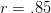$r=.85$! Why? What do you mean why? This is the most natural choice!”

This number is a made-up parameter in the sense that there is no justification for choosing it over another value.

Of course, if the ordering that PageRank produces is independent from the choice of$r$ then we are OK.

When I tried to find such a theorem, I got nothing. Then I started to realize that there can’t be such a theorem. First, if there was, the only reason to choose a number over another would be the speed of convergence of the algorithm (.84 is better than .85). Second, the “naive” PageRank (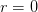$r=0$) could serve as a counterexample. To confirm my hunch I did some literature search and quickly discovered this paper (and others): Bressan and Peserico Choose the damping, choose the ranking? J. Discrete Algorithms 8 (2010), no. 2, 199–213. To quote:

“We prove that, at least on some graphs, the top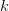$k$ nodes assume all possible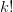$k!$ orderings as the damping factor varies, even if it varies within an arbitrarily small interval (e.g. [0.84999, 0.85001]).”

PageRank is often described as “elegant”. However, pulling a parameter from your ear is not only a very poor taste mathematically, it’s bad science. It’s similar to creating a mathematical theory of, say, planetary motion and deciding that the gravitational constant should be equal to, well,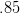$.85$. And why not? You do get a valid mathematical theory! Or, how about$\pi$ equal to$.85$?

There are of course other problems with PageRank. For example, there is an example of such a graph that changing a single link completely reverses the ranking (Ronny Lempel, Shlomo Moran: Rank-Stability and Rank-Similarity of Link-Based Web Ranking Algorithms in Authority-Connected Graphs. Inf. Retr. 8(2): 245-264 (2005)).

## Consequences of bad math

There is a lot of mathematics in computational science, algorithms, software etc. It’s tempting to make math stuff up just because you can. The consequences are dare.

There is nothing more practical than a good theory. Conversely, a bad theory makes things based on it very impractical.

This is you: “My car is great: a powerful engine, excellent mileage, leather interior, etc. I love it!”You don’t mind the bumpy ride? “What bumpy ride? Aren’t all cars supposed to run like this?”So, you haven’t figured out the right shape (the math) for your wheels and you pay the price.

## Worldwide suffering

Wikipedia: “the PageRank concept has proven to be vulnerable to manipulation, and extensive research has been devoted to identifying falsely inflated PageRank and ways to ignore links from documents with falsely inflated PageRank.”

In particular, (semi-)closed communities represent a problem. Linkfarms is the main example. Also, groups of hotels have a lot links to each other, but very few links to the rest of the web. The issue is that once the random surfer gets into one of such communities he can’t get out, adding more and more to the community’s PageRank.

Finding such communities of web pages, if they try to avoid detection, is an NP-hard problem (read “hard”). The answer from Google and others is to employ secret heuristics that identify these communities and then penalize their PageRank.

The result is numerous false positives that hurt legitimate sites and false negatives that rank linkfarms higher in the search results. In addition, the secrecy and the constant tweaking make the risks unmanageable for web-based businesses. It’s a mess.

To summarize, this is the sequence of events:

• the initial model produces meaningless results;
• they come up with an ad hoc fix;
• the model is still mathematically bad;
• the model becomes dominant nonetheless;
• now we’re all screwed.

There will always be bad math out there. What makes this a real abomination is the magnitude of the problem, i.e., Google. As a mathematician I find it disturbing that one piece of bad mathematics affects just about every Internet user in the world.

Did I justify the harsh language that I used here? You’ll be the judge of that.

Meanwhile what is the right way of ranking sites based on links? I’ll write a post about that in the near future. Short version: remove all “cycles”.

This is an excerpt from a longer article about PageRank.

Follow-ups: here and here.